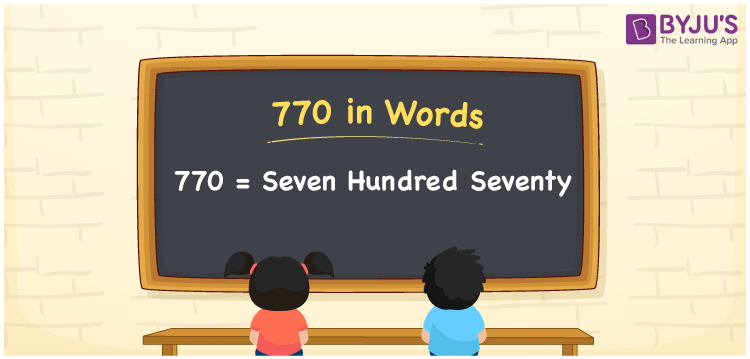# 770 in Words

We can write 770 in words as Seven hundred seventy. We use the word form of numbers to spell or express a certain quantity or measures. For example, to express or tell the price of a toy, say Rs. 770, we can say, “The price of the toy is Seven hundred seventy rupees”. In this article, you will learn how to derive the number 770 in word form using a place value chart and some interesting facts about 770.

 770 in words Seven hundred seventy Seven hundred seventy in Numbers 770

## 770 in English Words

We generally use the English alphabet to express numbers in words. Thus, we can read 770 in English words as “Seven hundred seventy”.## How to Write 770 in Words?

Let’s learn how to write the number 770 in words with the help of a below place value chart for 770.

 Hundreds Tens Ones 7 7 0

Here, ones = 0, tens = 7, hundreds = 7

These digits can be expanded as follows.

7 × Hundred + 7 × Ten + 0 × One

= 7 × 100 + 7 × 10

= 700 + 70

= Seven hundred + Seventy

= Seven hundred seventy

Thus, 770 in words = Seven hundred seventy

We know that 770 is a natural number that precedes 771 and succeeds 769.

770 in words – Seven hundred seventy

Is 770 an even number? – Yes

Is 770 an odd number? – No

Is 770 a prime number? – No

Is 770 a composite number? – Yes

Is 770 a perfect square number? – No

Is 770 a perfect cube number? – No

## Frequently Asked Questions on 770 in Words

Q1

### How do you spell 770 in words?

We can spell the number 770 using the English words as Seven hundred seventy.
Q2

### What is the number name of 770?

The number name of 770 is Seven hundred seventy.
Q3

### How to write Rs. 770 in words on a cheque?

On a cheque, we can write Rs. 770 in words as “Seven hundred seventy rupees only”.